# gkrecall

We brings you daily Current affairs, daily Current Affairs Quiz, weekly Current Affairs, weekly Current Affairs Quiz to enhance your preparation for upcoming exams. We also provide articles related to quant, reasoning, English, ssc etc .

##RRB Clerk and RRB PO Prelims exam are going to be held in the upcoming months. GKRECALL have already provided you with the PDFs of many topics of QuantitativeAptitudelike NumberSeries,data interpretation.important, simplification,
Arithmetic questions to prepare for RRB Clerk and PO Pre exam. Practicing these questions will help you to know about the level of the questions. To increase your speed and accuracy, enhance your calculations.

DIRECTION :- The given bar graph shows the number of phones (Samsung, Micromax and MI) sold by Mobile House in different 5 different years. Study the graph and answer the following questions.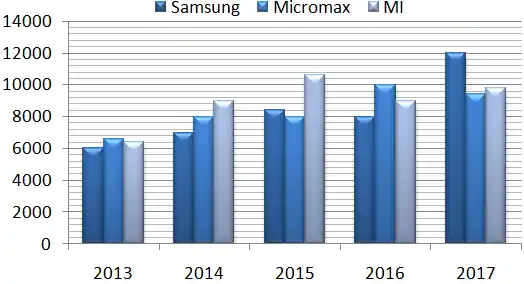1.Samsung phones sold in 2014 and 2013 together is what percent more/loss then Micromax phones sold in 2016 ?
A.137.5%
B.75%
C.30%
D.84%
E.80%

2.Find the difference between average number of Samsung phones sold to average number of MI mobile sold in given five years by Mobile House?
A.680
B.820
C.908
D.536
E.625

3.If Samsung, Micromax and MI phones sold in 2017 is 20%, 100% and 0% respectively more than these mobiles sold in 2018 respectively, then, find (approx) average number of phones sold in 2018 of all three companies by Mobile House ?
A.4000
B.6275
C.5600
D.8167
E.5000

4.In 2013, MI phones sold to male customers and female customers is in the ratio 7 : 9. In 2014, MI phones sold to male customers and female customers in the ratio 4 : 5. Find the ratio of male customers to female customers who bought MI in 2013 and 2014 together ?
A.17 : 13
B.34 : 43
C.31 : 19
D.11 : 15
E.None of these

5.If in 2013, 10.53 % customer of Mobile House are female and male customer to female customer ratio of Samsung and MI in 2013 is 5 : 1 and 7 : 1 respectively then, find the female customers who bought Micromax phones in 2013.
A.200
B.350
C.600
D.540
E.480
6.Ram interchanged positions of the digits of a two-digit number, the number obtained is smaller than the original number by 36. If the digits of the number are in the ratio of 2 : 4, what is the original number ?
A.24
B.85
C.84
D.63
E.None of these
7.A jar full of whisky contains 50% alcohol. A part of this whisky is replaced by another containing 25% alcohol and now the percentage of alcohol was found to be 30%. The quantity of whisky replaced is :

A1/3
B2/3
C4/5
D3/5
ENone of these

8.3xy5’ is a four digit number then ‘xy’ is?
Statement I: xy is exactly divisible by 23
Statement II: 3xy5 is exactly divisible by 25
1. If the data in statement I alone is sufficient to answer the question
2. If the data in statement II alone is sufficient to answer the question
3. If the data either in Statement I alone or Statement II alone are sufficient to answer the question
4. If the data given in Both Statements I & II are not sufficient to answer the question
5. If the data given in both statements I & II together are necessary to answer the question

9.a,b are integers, Is b exactly divisible by 20?
Statement I: a/20 + b/20 is an integer
Statement II: a/7 + b/20 is an integer
1. If the data in statement I alone is sufficient to answer the question
2. If the data in statement II alone is sufficient to answer the question
3. If the data either in Statement I alone or Statement II alone are sufficient to answer the question
4. If the data given in Both Statements I & II are not sufficient to answer the question
5. If the data given in both statements I & II together are necessary to answer the question

10.Is 12 is one of the number in 13 consecutive even numbers.
Statement I: Difference between first and least numbers is 24.
Statement II: Average of the set is zero.
1. If the data in statement I alone is sufficient to answer the question
2. If the data in statement II alone is sufficient to answer the question
3. If the data either in Statement I alone or Statement II alone are sufficient to answer the question
4. If the data given in Both Statements I & II are not sufficient to answer the question
5. If the data given in both statements I & II together are necessary to answer the question

SOLUTIONS

1.Required Percentage =*100 = 30%

2.Average Samsung phones sold =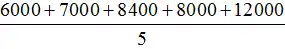= 8280
Average of Mi phone sold =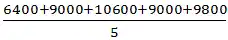= 8960
Required difference = 680

3.Samsung phone sold in 2018 = 12000*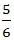= 10000
Micromax phone sold in 2018 =  9400/2 = 4700
MI phones sold in 2012 = 9800
Required average =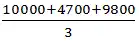= 8167(approx)

4.Solution:
Required ratio =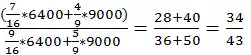5.Total customers = 19000
total female customers = 2/19*19000=2000
Females who bought Micromax phones
= 2000-(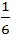*6000 +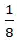*6400))
=2000-(1000 + 800) = 200

6.Let the number be 10x+y
After interchanging digits, the number will be 10y+x
As given, 10x+y-10y-x=36
⇒x-y = 4
Since, 2x = 4y
Or, x = 2y
Therefore, x=8 y = 4
And hence the required number is 84

7.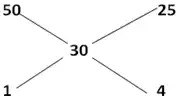8.Answer – 5. If the data given in both statements I & II together are necessary to answer the question
Explanation :
from statement I: xy could be 23, 46, 69,92
3xy5 will be 3235,3465,3695,3925
3xy5 divisible by 25
only 3925/25 is exactly divisible

9.Answer – 2. If the data in statement II alone is sufficient to answer the question
Explanation :
second statement to be integer definitely ‘a’ must be multiple of 7 let it be 140 then b must be multiple of 20 to give integer.

10.Answer – 2. If the data in statement II alone is sufficient to answer the question
Explanation :
Average of the set is zero: in that case 12 must be there in the set